# A.1.4.2 Practice Describing Distributions

Choose which type(s) of distribution describe the dot plot.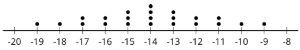Select all that apply
• A
• B
• C
• D
• E
• F

Choose which type(s) of distribution describe the dot plot.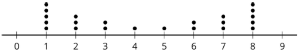Select all that apply
• A
• B
• C
• D
• E
• F

Choose which type(s) of distribution describe the dot plot.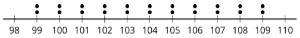Select all that apply
• A
• B
• C
• D
• E
• F

Choose which type(s) of distribution describe the dot plot.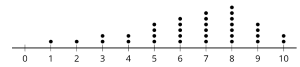Select all that apply
• A
• B
• C
• D
• E
• F

Choose which type(s) of distribution describe the dot plot.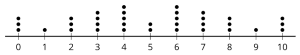Select all that apply
• A
• B
• C
• D
• E
• F

Choose which type(s) of distribution describe the dot plot.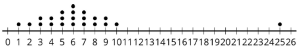Select all that apply
• A
• B
• C
• D
• E
• F

Choose which type(s) of distribution describe the dot plot.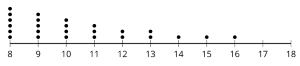Select all that apply
• A
• B
• C
• D
• E
• F